# Frank Solutions Class 9 Maths Chapter 21 Areas Theorems on Parallelograms

Frank Solutions Class 9 Maths Chapter 21 Areas Theorems on Parallelograms provide comprehensive answers to each and every question of textbook problems. Students who are in search of the best study source are recommended to follow Frank Solutions, as they help them to enhance their conceptual knowledge. Regular practice of these solutions improves time management and problem-solving skills, which are necessary to boost academic performance. Here, students can avail of Frank Solutions Class 9 Maths Chapter 21 Areas Theorems on Parallelograms from the below-mentioned link.

Chapter 21 deals with the areas of parallelograms. A region covered by a parallelogram in a two-dimensional plane is known as the area of a parallelogram. The solutions are designed in a lucid manner, based on students’ understanding capacity. The geometrical figures help students to grasp the concepts with ease.

## Frank Solutions for Class 9 Maths Chapter 21 Areas Theorems on Parallelograms – Download the PDF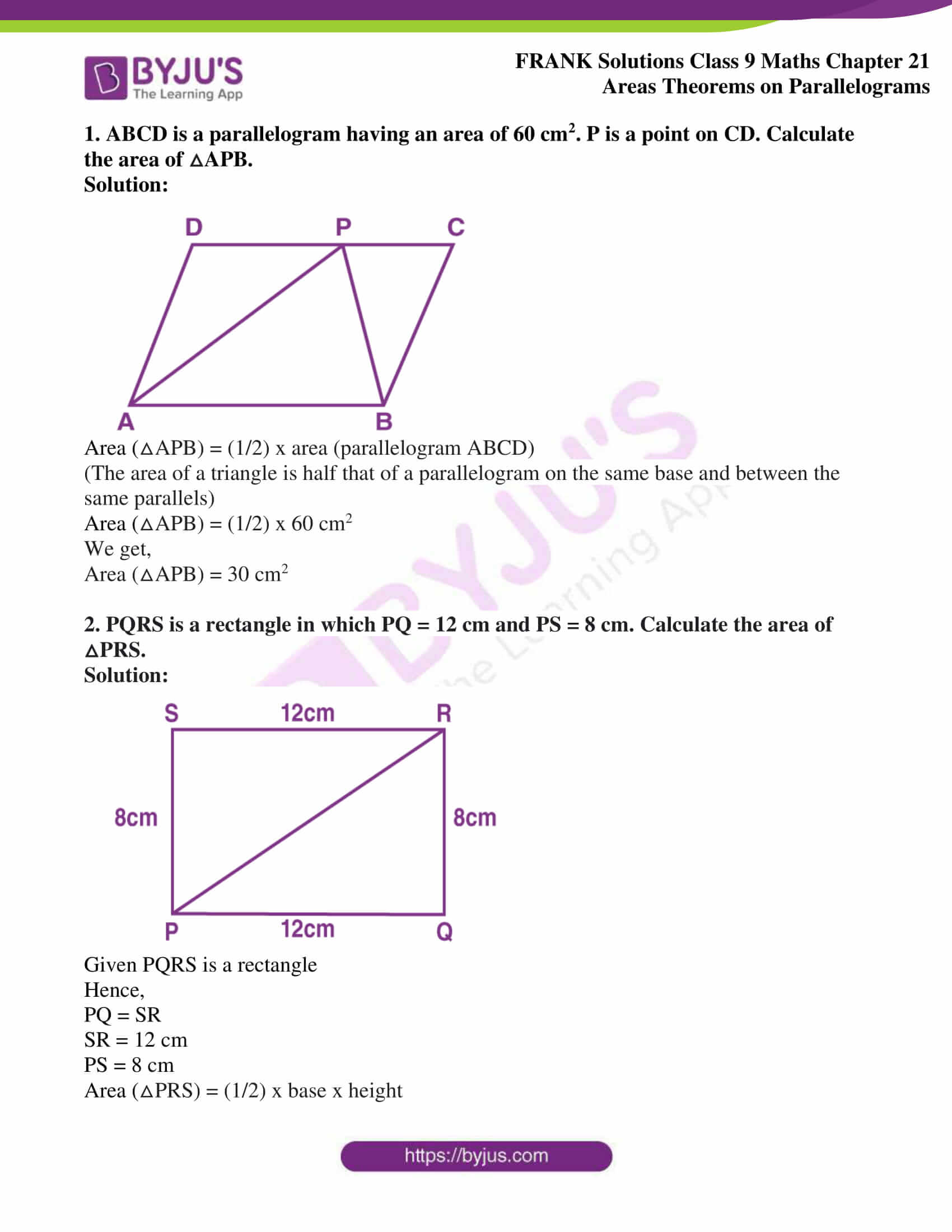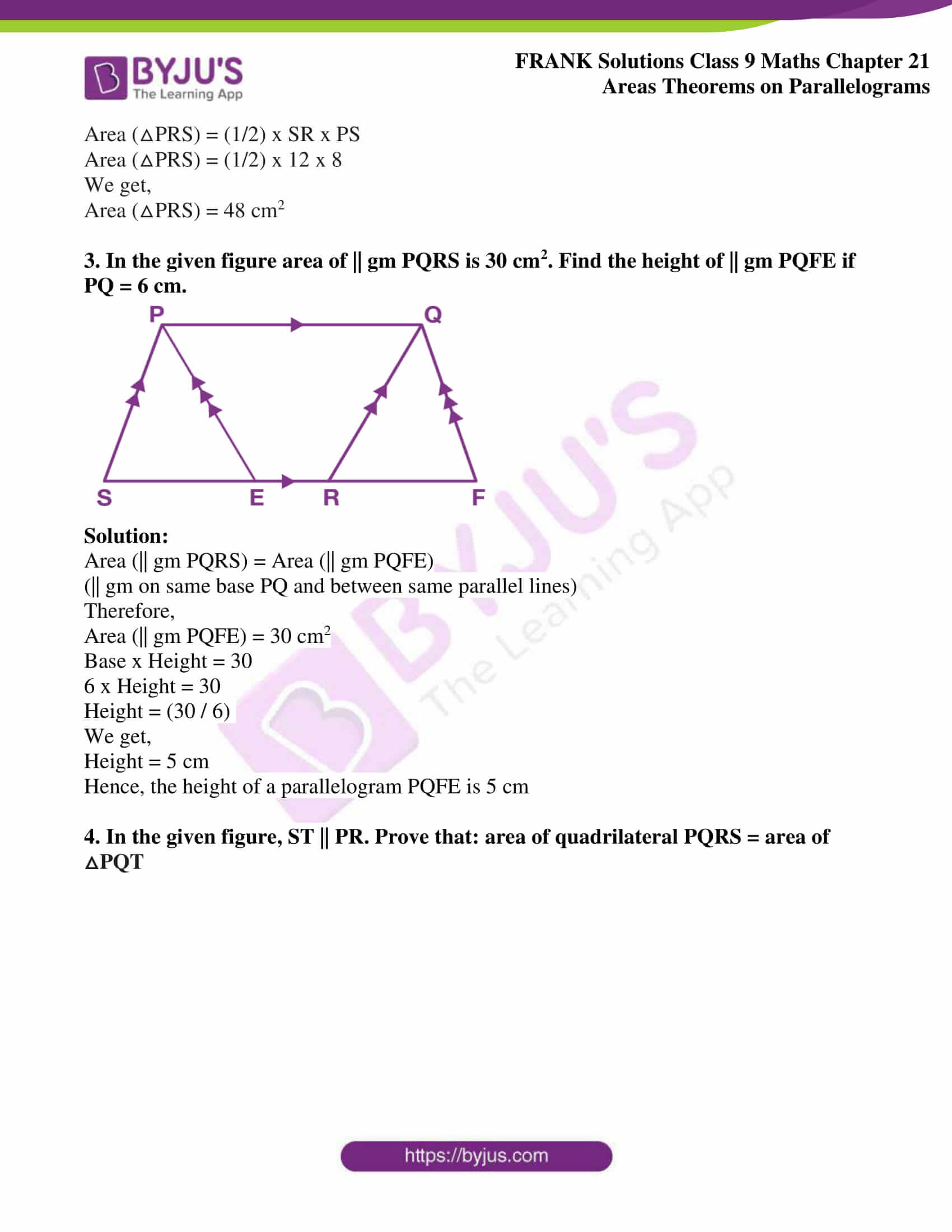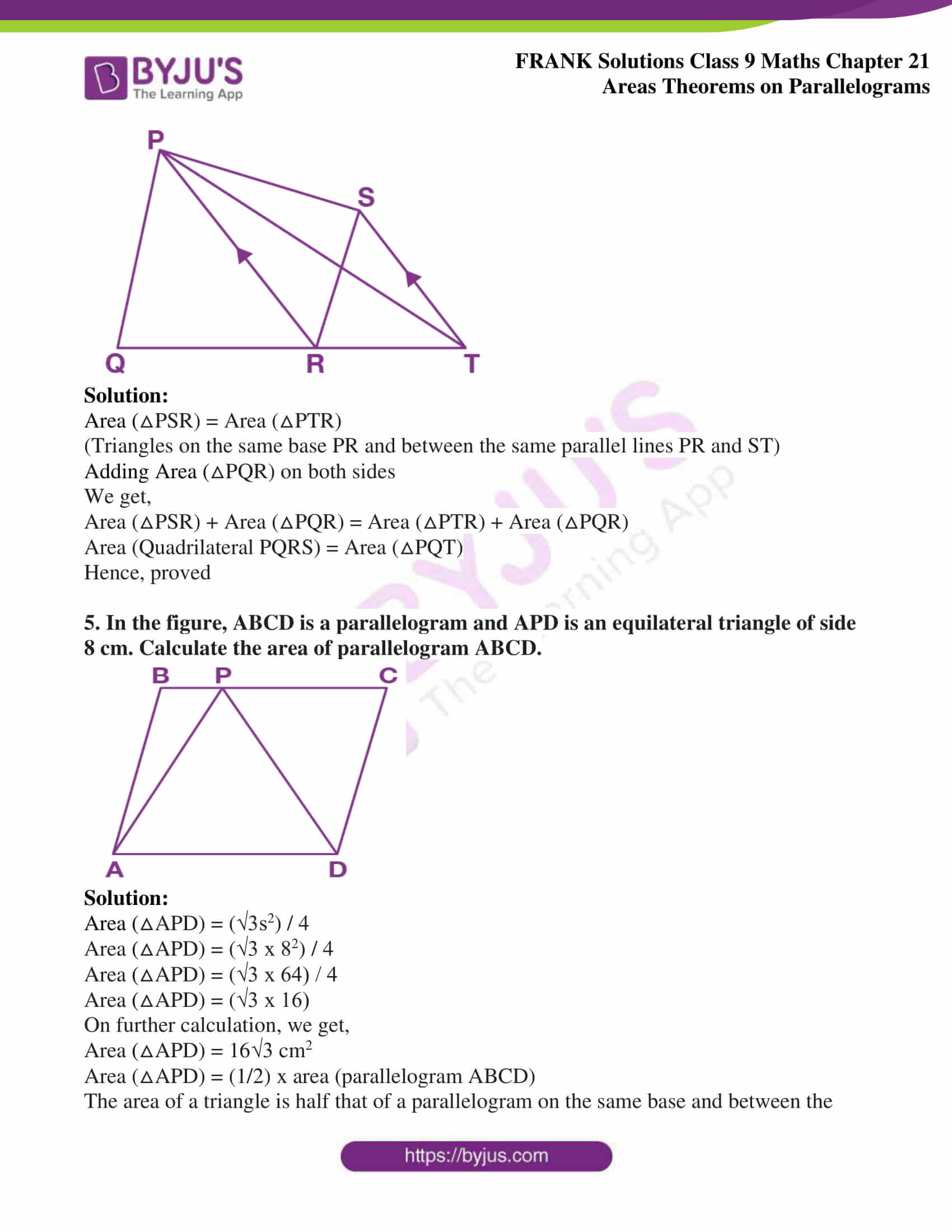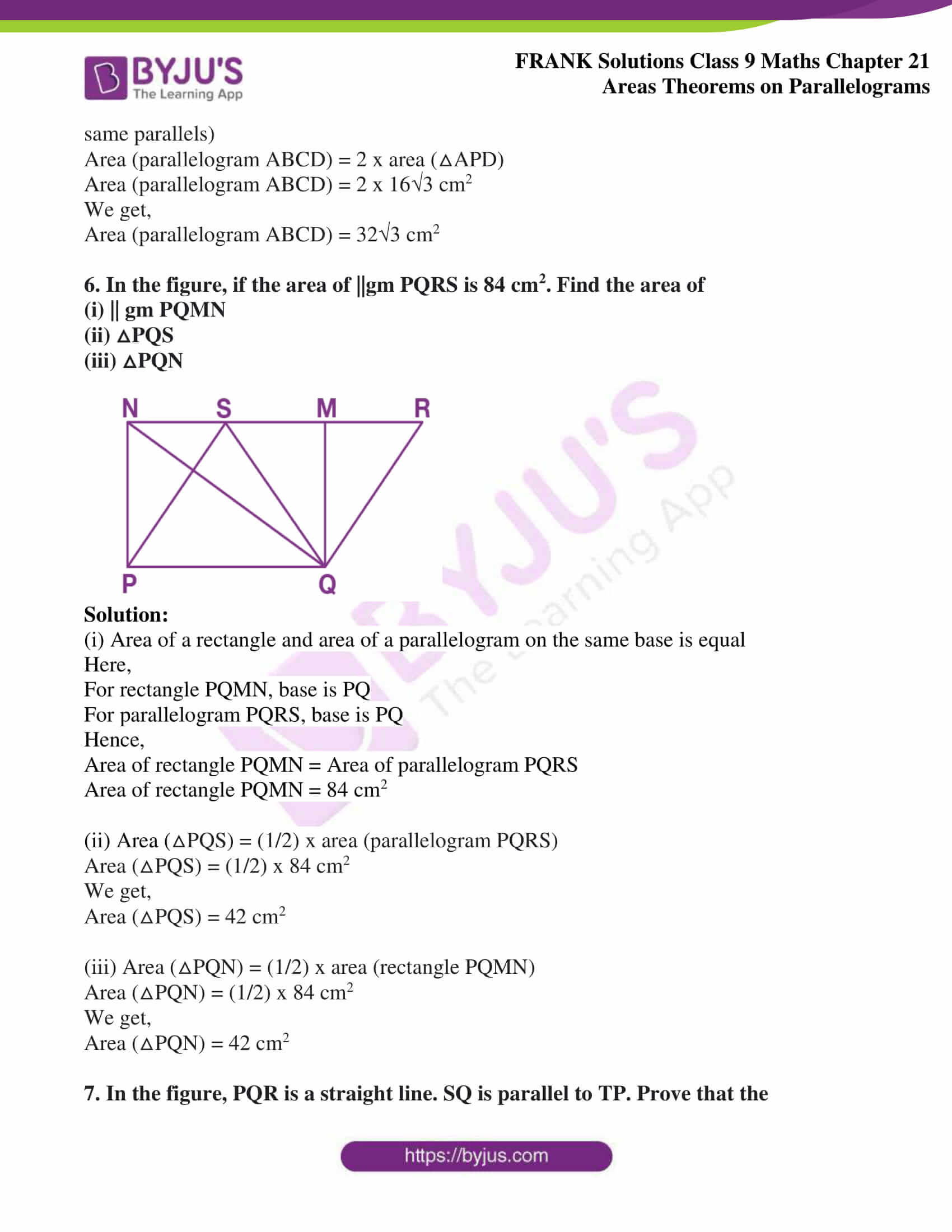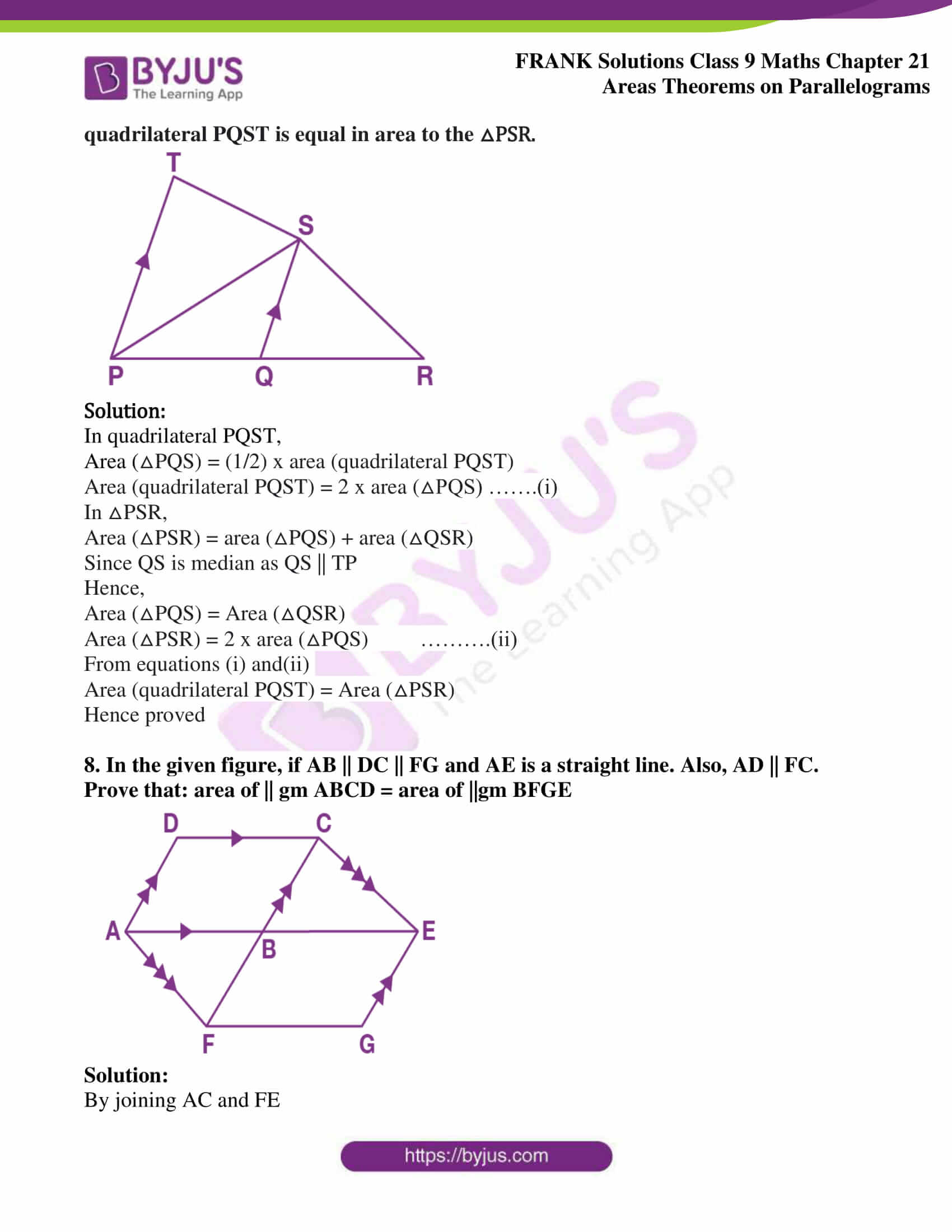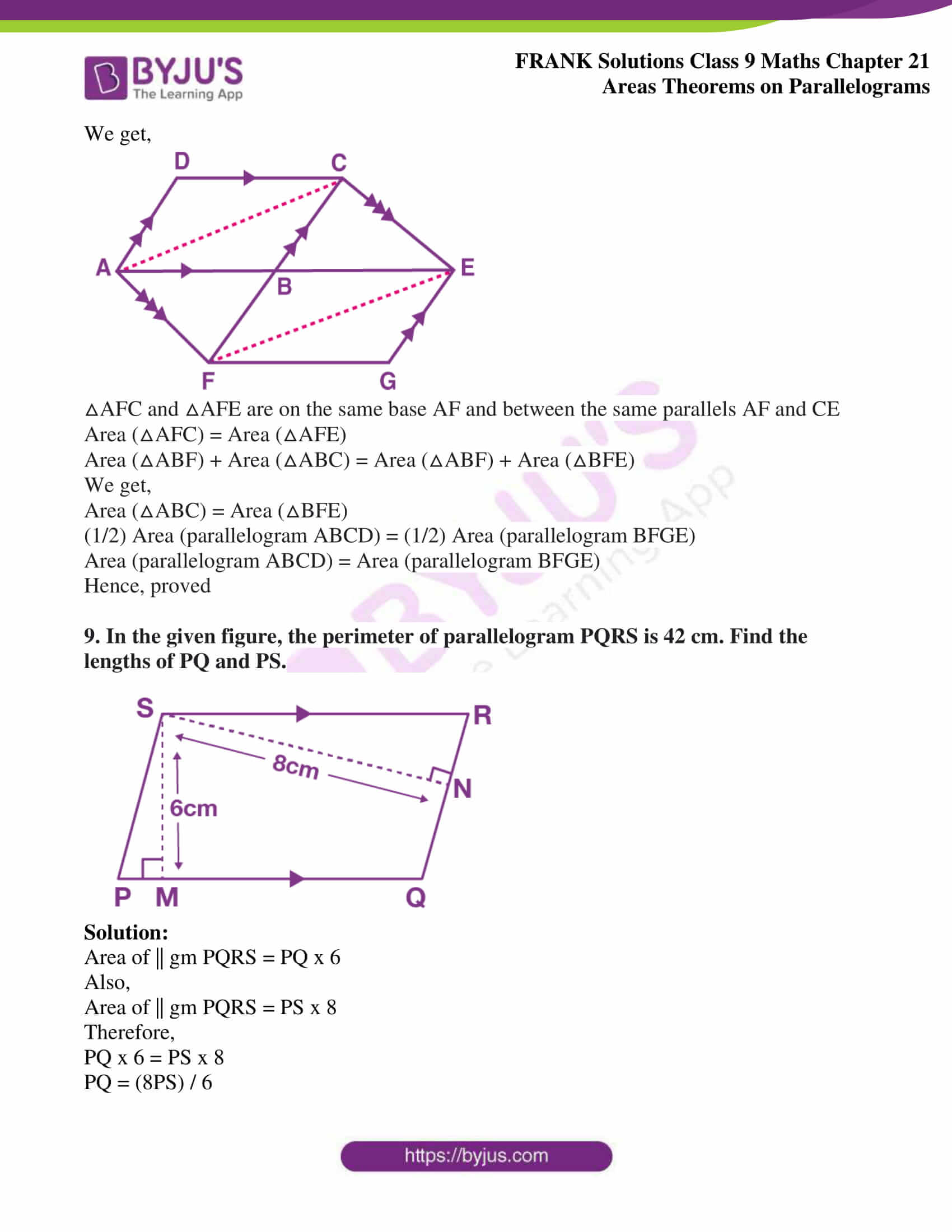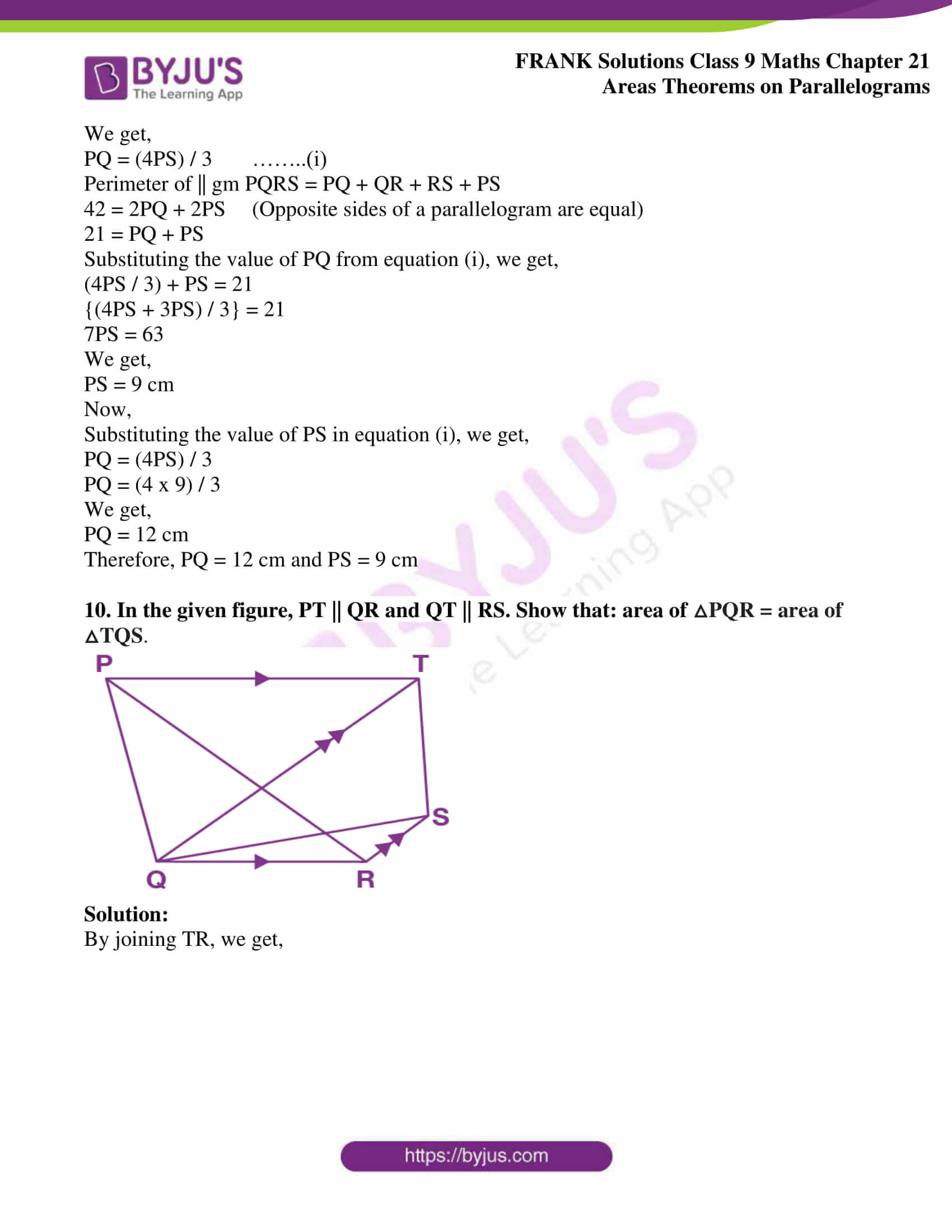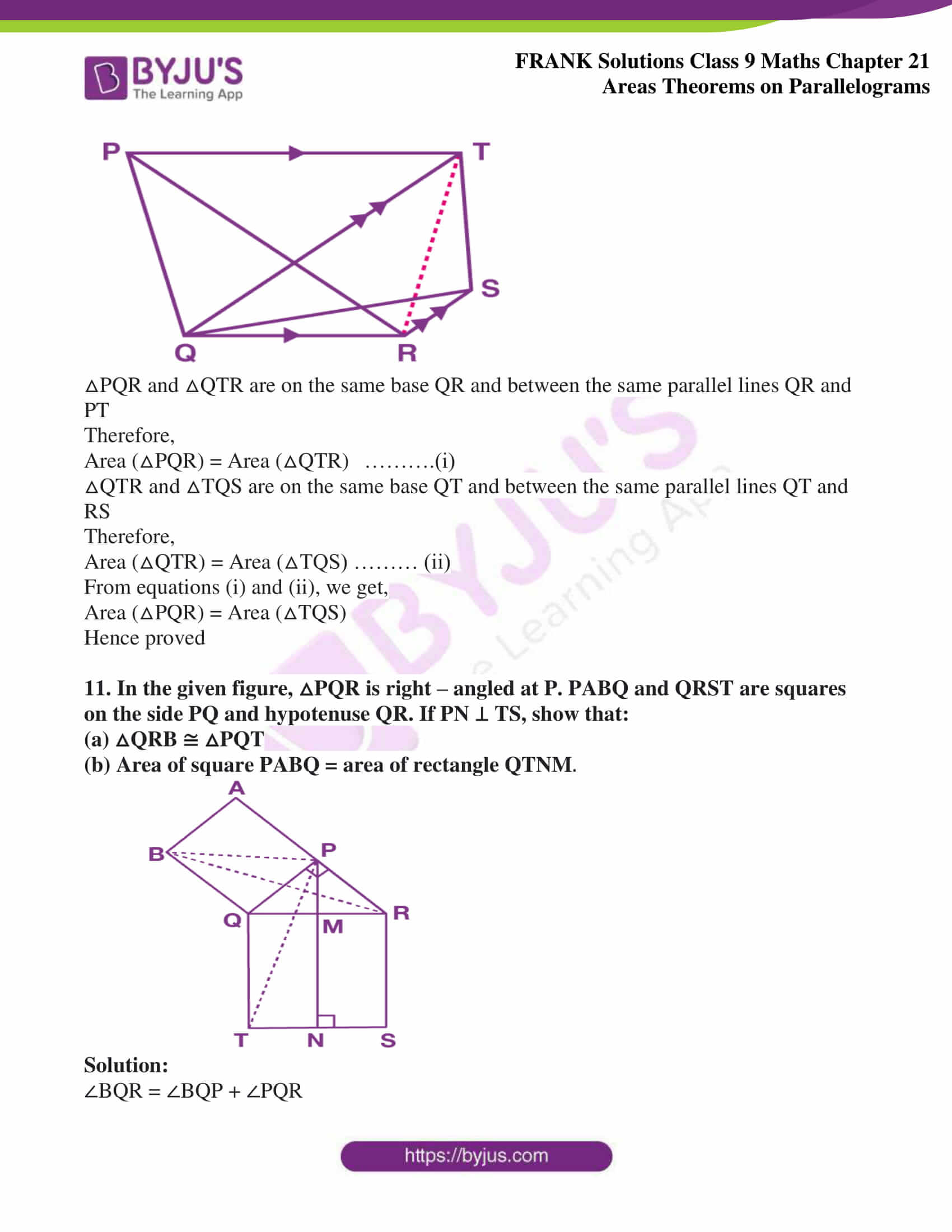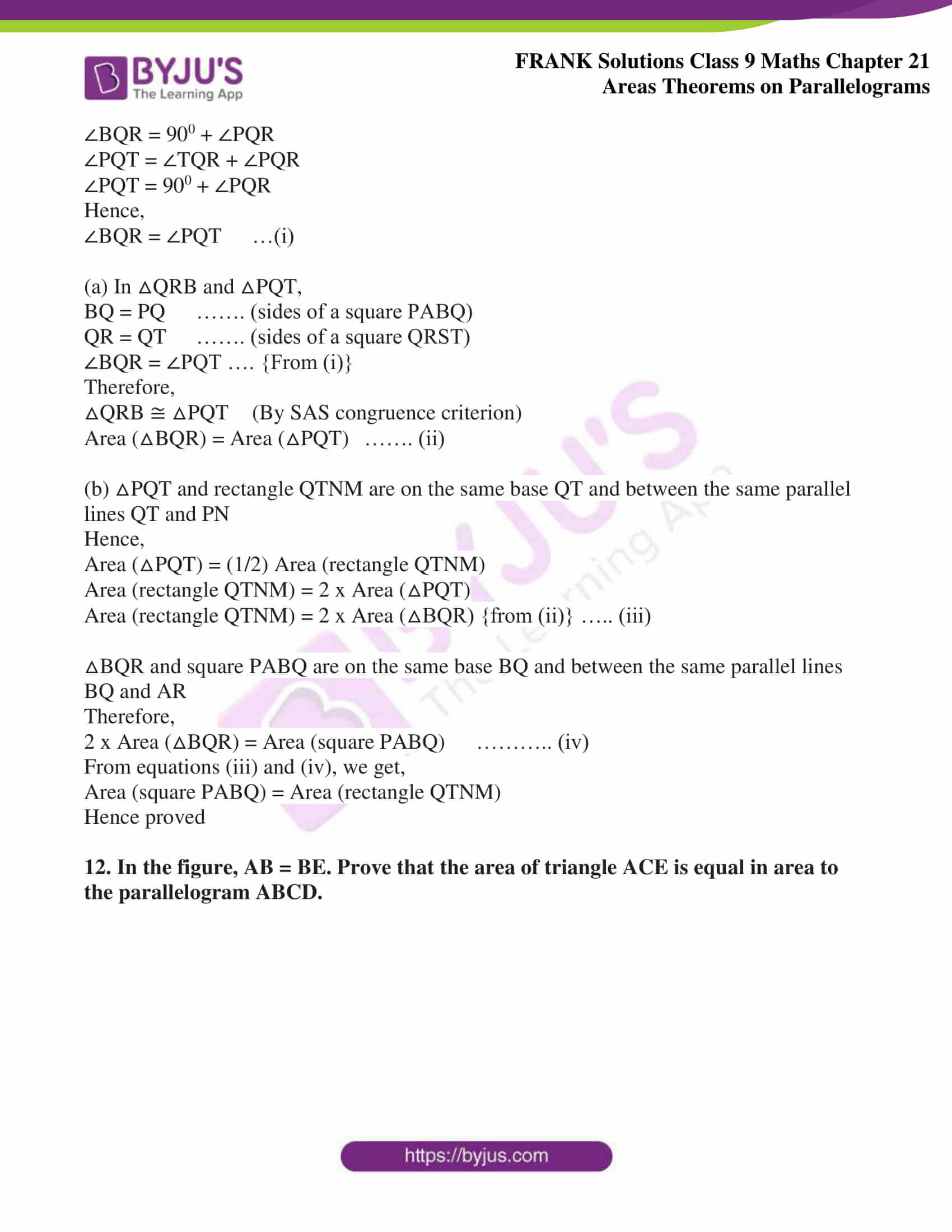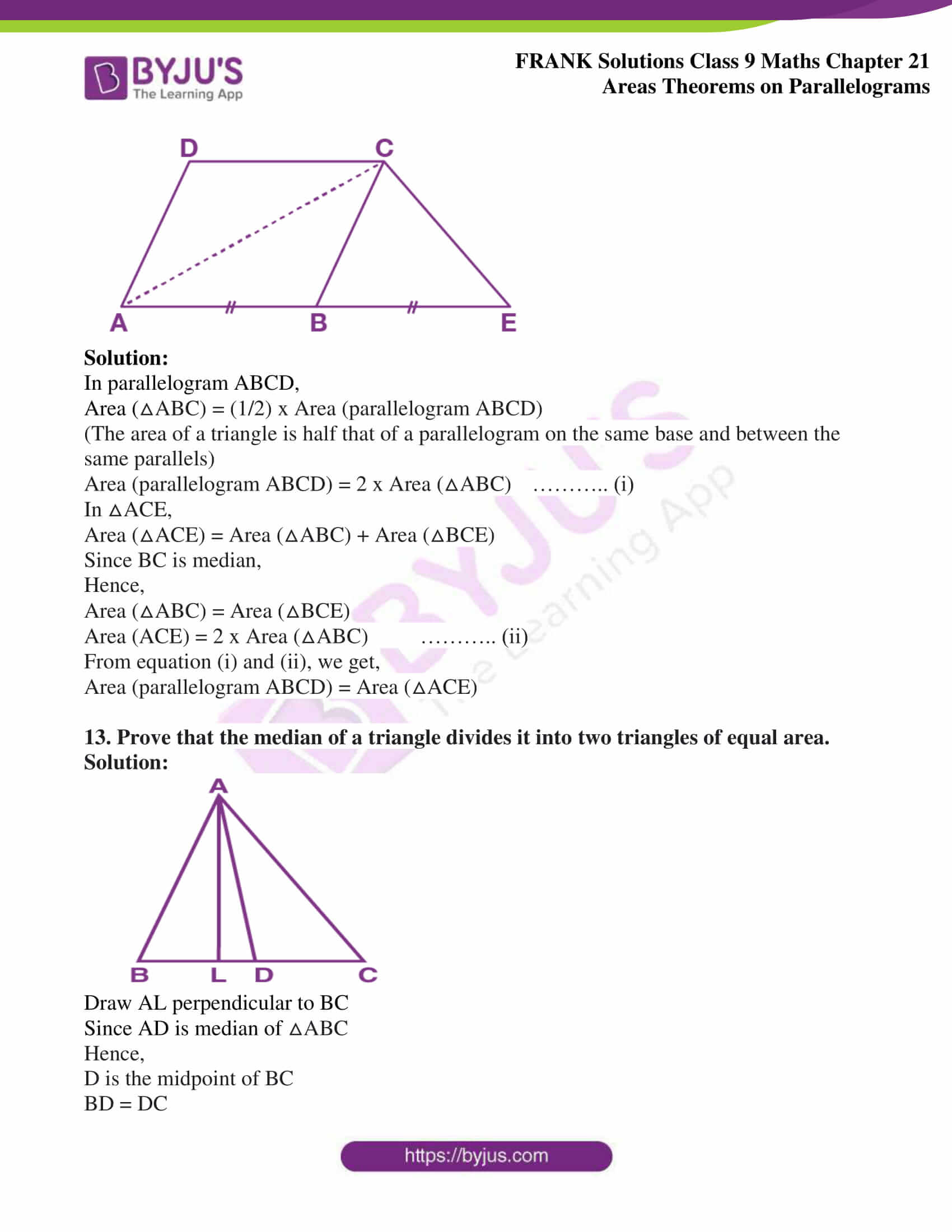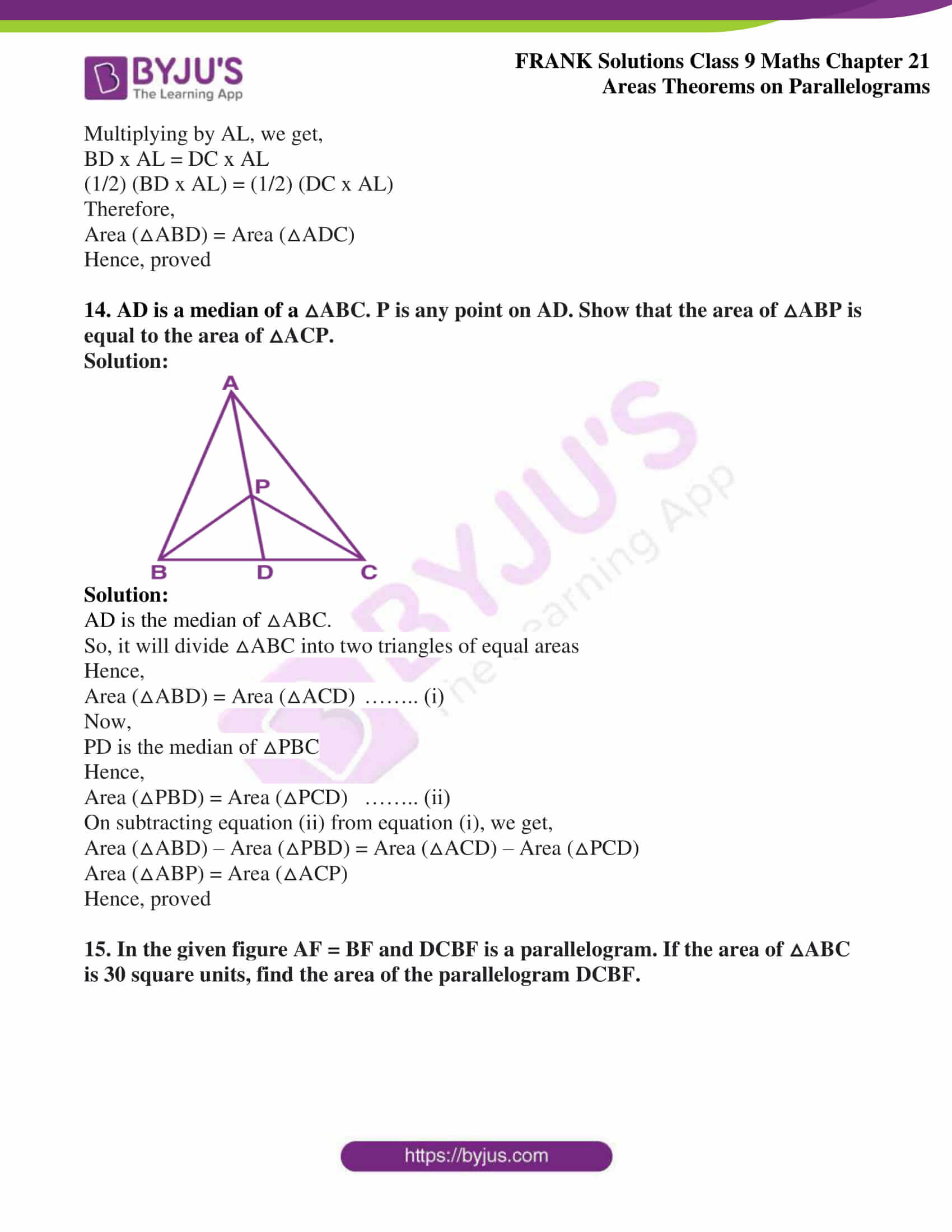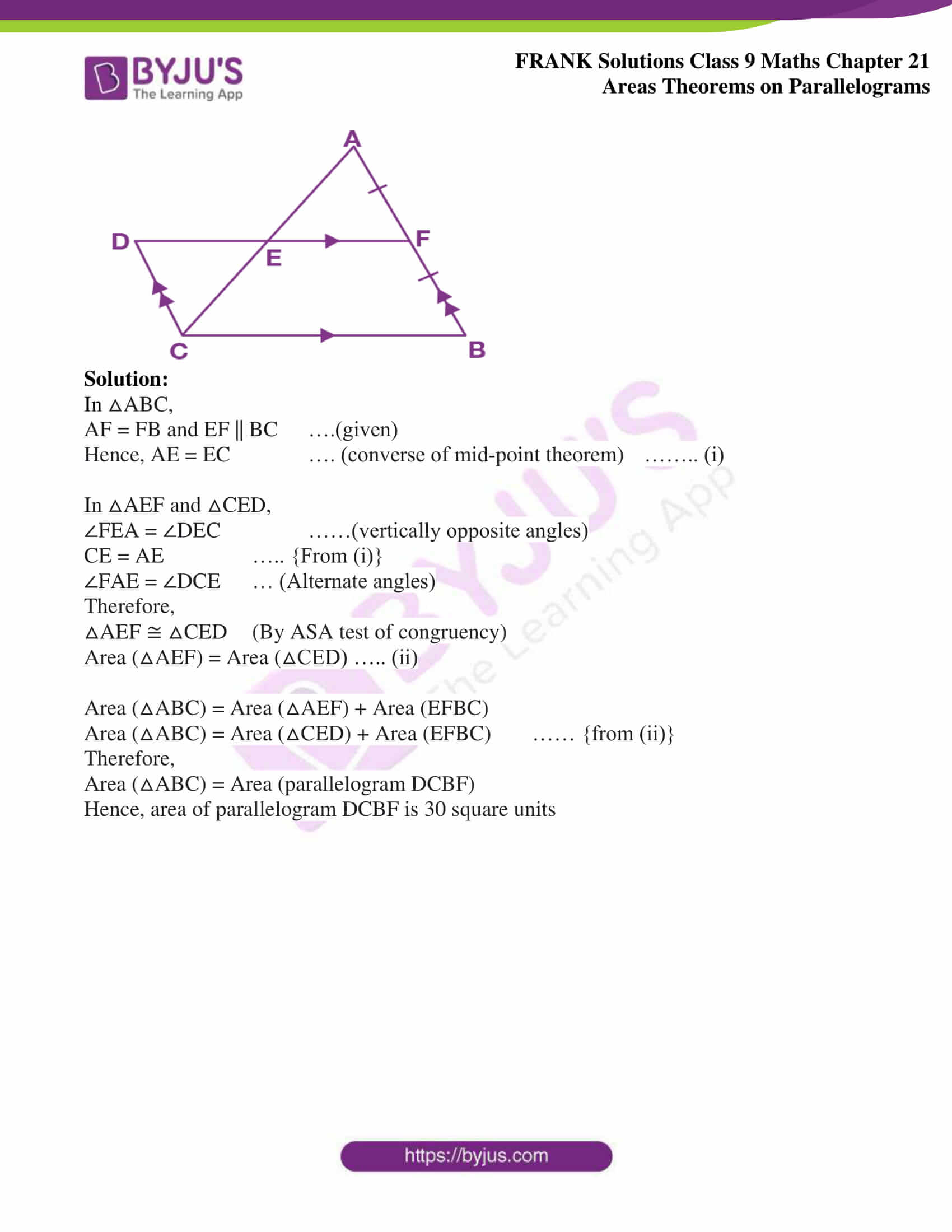## Access Frank Solutions for Class 9 Maths Chapter 21 Areas Theorems on Parallelograms

1. ABCD is a parallelogram having an area of 60 cm2. P is a point on CD. Calculate the area of △APB.

Solution: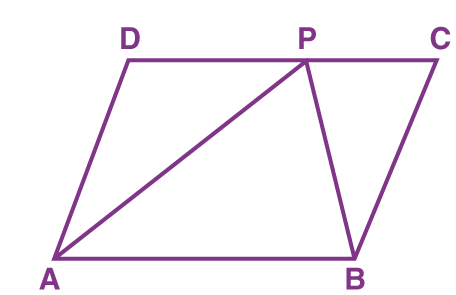Area (△APB) = (1/2) x area (parallelogram ABCD)

(The area of a triangle is half that of a parallelogram on the same base and between the same parallels)

Area (△APB) = (1/2) x 60 cm2

We get,

Area (△APB) = 30 cm2

2. PQRS is a rectangle in which PQ = 12 cm and PS = 8 cm. Calculate the area of △PRS.

Solution: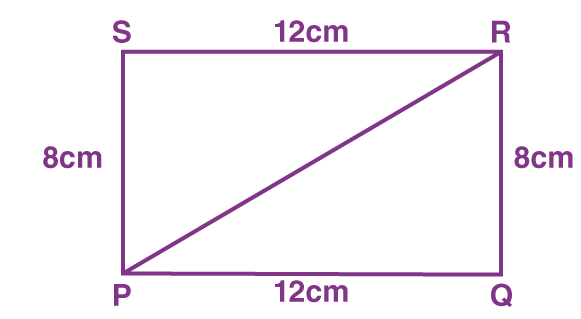Given PQRS is a rectangle

Hence,

PQ = SR

SR = 12 cm

PS = 8 cm

Area (△PRS) = (1/2) x base x height

Area (△PRS) = (1/2) x SR x PS

Area (△PRS) = (1/2) x 12 x 8

We get,

Area (△PRS) = 48 cm2

3. In the given figure area of || gm PQRS is 30 cm2. Find the height of || gm PQFE if PQ = 6 cm.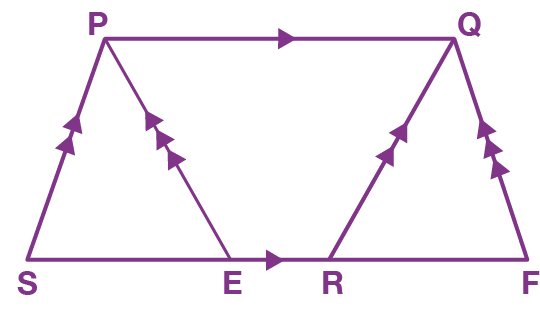Solution:

Area (|| gm PQRS) = Area (|| gm PQFE)

(|| gm on same base PQ and between same parallel lines)

Therefore,

Area (|| gm PQFE) = 30 cm2

Base x Height = 30

6 x Height = 30

Height = (30 / 6)

We get,

Height = 5 cm

Hence, the height of a parallelogram PQFE is 5 cm

4. In the given figure, ST || PR. Prove that: area of quadrilateral PQRS = area of △PQT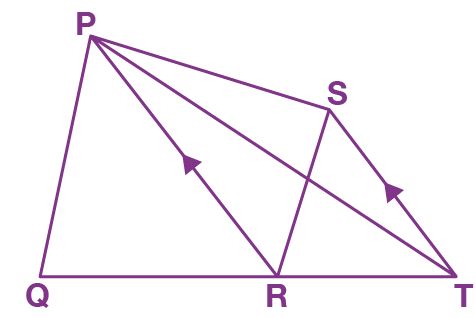Solution:

Area (△PSR) = Area (△PTR)

(Triangles on the same base PR and between the same parallel lines PR and ST)

Adding Area (△PQR) on both sides

We get,

Area (△PSR) + Area (△PQR) = Area (△PTR) + Area (△PQR)

Area (Quadrilateral PQRS) = Area (△PQT)

Hence, proved

5. In the figure, ABCD is a parallelogram and APD is an equilateral triangle of side 8 cm. Calculate the area of parallelogram ABCD.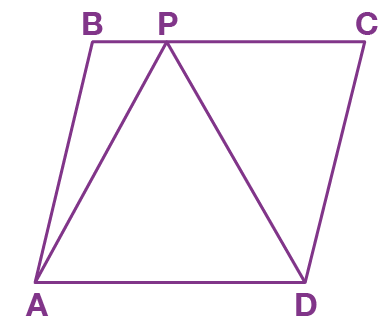Solution:

Area (△APD) = (√3s2) / 4

Area (△APD) = (√3 x 82) / 4

Area (△APD) = (√3 x 64) / 4

Area (△APD) = (√3 x 16)

On further calculation, we get,

Area (△APD) = 16√3 cm2

Area (△APD) = (1/2) x area (parallelogram ABCD)

The area of a triangle is half that of a parallelogram on the same base and between the same parallels)

Area (parallelogram ABCD) = 2 x area (△APD)

Area (parallelogram ABCD) = 2 x 16√3 cm2

We get,

Area (parallelogram ABCD) = 32√3 cm2

6. In the figure, if the area of ||gm PQRS is 84 cm2. Find the area of

(i) || gm PQMN

(ii) △PQS

(iii) △PQN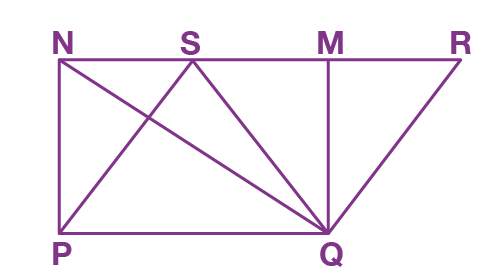Solution:

(i) Area of a rectangle and area of a parallelogram on the same base is equal

Here,

For rectangle PQMN, base is PQ

For parallelogram PQRS, base is PQ

Hence,

Area of rectangle PQMN = Area of parallelogram PQRS

Area of rectangle PQMN = 84 cm2

(ii) Area (△PQS) = (1/2) x area (parallelogram PQRS)

Area (△PQS) = (1/2) x 84 cm2

We get,

Area (△PQS) = 42 cm2

(iii) Area (△PQN) = (1/2) x area (rectangle PQMN)

Area (△PQN) = (1/2) x 84 cm2

We get,

Area (△PQN) = 42 cm2

7. In the figure, PQR is a straight line. SQ is parallel to TP. Prove that the quadrilateral PQST is equal in area to the △PSR.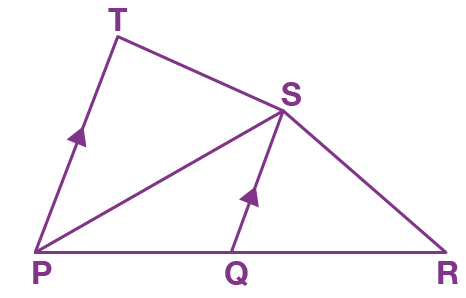Solution:

Area (△PQS) = (1/2) x area (quadrilateral PQST)

Area (quadrilateral PQST) = 2 x area (△PQS) …….(i)

In △PSR,

Area (△PSR) = area (△PQS) + area (△QSR)

Since QS is median as QS || TP

Hence,

Area (△PQS) = Area (△QSR)

Area (△PSR) = 2 x area (△PQS) ……….(ii)

From equations (i) and(ii)

Area (quadrilateral PQST) = Area (△PSR)

Hence proved

8. In the given figure, if AB || DC || FG and AE is a straight line. Also, AD || FC. Prove that: area of || gm ABCD = area of ||gm BFGESolution:

By joining AC and FE

We get,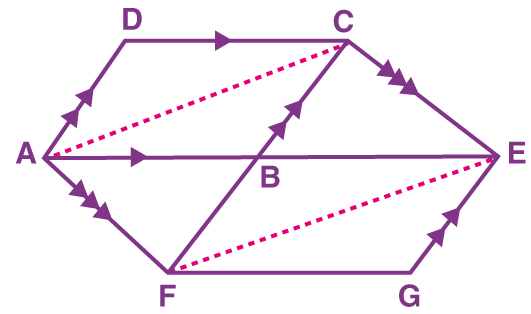△AFC and △AFE are on the same base AF and between the same parallels AF and CE

Area (△AFC) = Area (△AFE)

Area (△ABF) + Area (△ABC) = Area (△ABF) + Area (△BFE)

We get,

Area (△ABC) = Area (△BFE)

(1/2) Area (parallelogram ABCD) = (1/2) Area (parallelogram BFGE)

Area (parallelogram ABCD) = Area (parallelogram BFGE)

Hence, proved

9. In the given figure, the perimeter of parallelogram PQRS is 42 cm. Find the lengths of PQ and PS.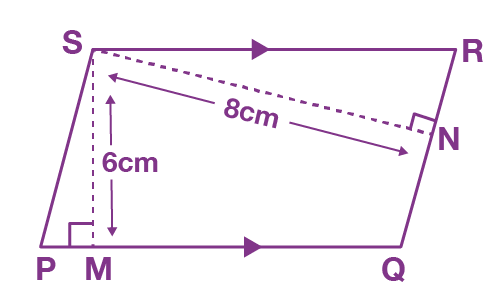Solution:

Area of || gm PQRS = PQ x 6

Also,

Area of || gm PQRS = PS x 8

Therefore,

PQ x 6 = PS x 8

PQ = (8PS) / 6

We get,

PQ = (4PS) / 3 ……..(i)

Perimeter of || gm PQRS = PQ + QR + RS + PS

42 = 2PQ + 2PS (Opposite sides of a parallelogram are equal)

21 = PQ + PS

Substituting the value of PQ from equation (i), we get,

(4PS / 3) + PS = 21

{(4PS + 3PS) / 3} = 21

7PS = 63

We get,

PS = 9 cm

Now,

Substituting the value of PS in equation (i), we get,

PQ = (4PS) / 3

PQ = (4 x 9) / 3

We get,

PQ = 12 cm

Therefore, PQ = 12 cm and PS = 9 cm

10. In the given figure, PT || QR and QT || RS. Show that: area of △PQR = area of △TQS.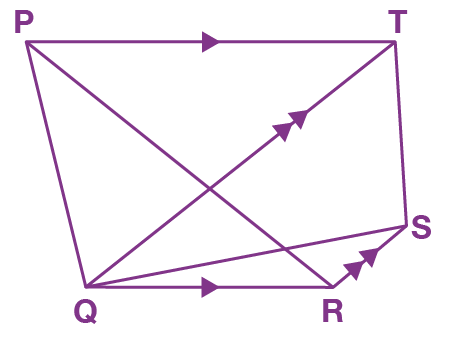Solution:

By joining TR, we get,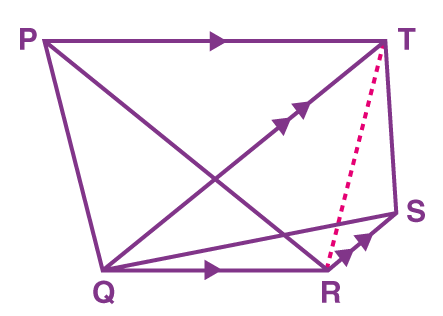△PQR and △QTR are on the same base QR and between the same parallel lines QR and PT

Therefore,

Area (△PQR) = Area (△QTR) ……….(i)

△QTR and △TQS are on the same base QT and between the same parallel lines QT and RS

Therefore,

Area (△QTR) = Area (△TQS) ……… (ii)

From equations (i) and (ii), we get,

Area (△PQR) = Area (△TQS)

Hence proved

11. In the given figure, △PQR is right – angled at P. PABQ and QRST are squares on the side PQ and hypotenuse QR. If PN ⊥ TS, show that:

(a) △QRB ≅ △PQT

(b) Area of square PABQ = area of rectangle QTNM.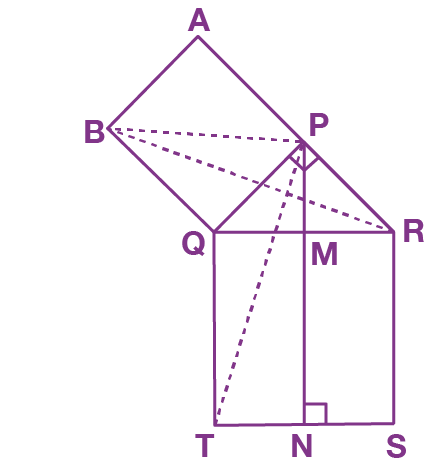Solution:

∠BQR = ∠BQP + ∠PQR

∠BQR = 900 + ∠PQR

∠PQT = ∠TQR + ∠PQR

∠PQT = 900 + ∠PQR

Hence,

∠BQR = ∠PQT …(i)

(a) In △QRB and △PQT,

BQ = PQ ……. (sides of a square PABQ)

QR = QT ……. (sides of a square QRST)

∠BQR = ∠PQT …. {From (i)}

Therefore,

△QRB ≅ △PQT (By SAS congruence criterion)

Area (△BQR) = Area (△PQT) ……. (ii)

(b) △PQT and rectangle QTNM are on the same base QT and between the same parallel lines QT and PN

Hence,

Area (△PQT) = (1/2) Area (rectangle QTNM)

Area (rectangle QTNM) = 2 x Area (△PQT)

Area (rectangle QTNM) = 2 x Area (△BQR) {from (ii)} ….. (iii)

△BQR and square PABQ are on the same base BQ and between the same parallel lines BQ and AR

Therefore,

2 x Area (△BQR) = Area (square PABQ) ……….. (iv)

From equations (iii) and (iv), we get,

Area (square PABQ) = Area (rectangle QTNM)

Hence proved

12. In the figure, AB = BE. Prove that the area of triangle ACE is equal in area to the parallelogram ABCD.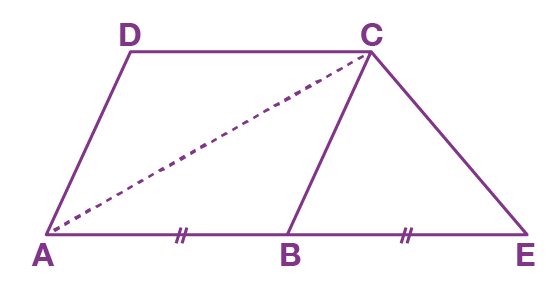Solution:

In parallelogram ABCD,

Area (△ABC) = (1/2) x Area (parallelogram ABCD)

(The area of a triangle is half that of a parallelogram on the same base and between the same parallels)

Area (parallelogram ABCD) = 2 x Area (△ABC) ……….. (i)

In △ACE,

Area (△ACE) = Area (△ABC) + Area (△BCE)

Since BC is median,

Hence,

Area (△ABC) = Area (△BCE)

Area (ACE) = 2 x Area (△ABC) ……….. (ii)

From equation (i) and (ii), we get,

Area (parallelogram ABCD) = Area (△ACE)

13. Prove that the median of a triangle divides it into two triangles of equal area.

Solution: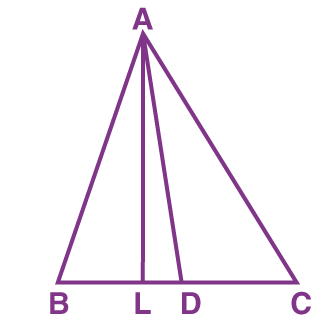Draw AL perpendicular to BC

Since AD is median of △ABC

Hence,

D is the midpoint of BC

BD = DC

Multiplying by AL, we get,

BD x AL = DC x AL

(1/2) (BD x AL) = (1/2) (DC x AL)

Therefore,

Hence, proved

14. AD is a median of a △ABC. P is any point on AD. Show that the area of △ABP is equal to the area of △ACP.

Solution: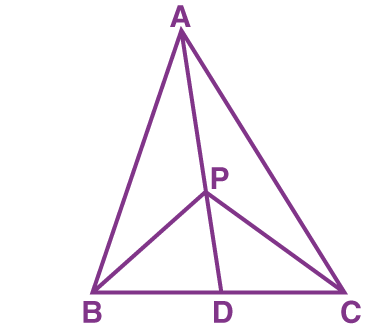Solution:

AD is the median of △ABC.

So, it will divide △ABC into two triangles of equal areas

Hence,

Area (△ABD) = Area (△ACD) …….. (i)

Now,

PD is the median of △PBC

Hence,

Area (△PBD) = Area (△PCD) …….. (ii)

On subtracting equation (ii) from equation (i), we get,

Area (△ABD) – Area (△PBD) = Area (△ACD) – Area (△PCD)

Area (△ABP) = Area (△ACP)

Hence, proved

15. In the given figure AF = BF and DCBF is a parallelogram. If the area of △ABC is 30 square units, find the area of the parallelogram DCBF.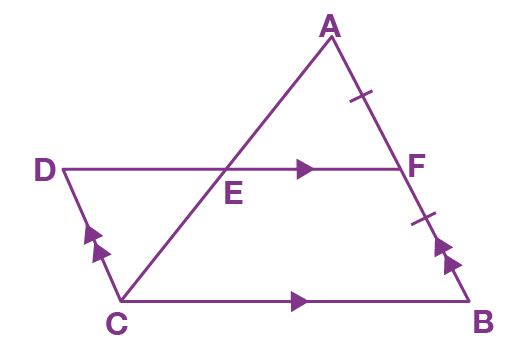Solution:

In △ABC,

AF = FB and EF || BC ….(given)

Hence, AE = EC …. (converse of mid-point theorem) …….. (i)

In △AEF and △CED,

∠FEA = ∠DEC ……(vertically opposite angles)

CE = AE ….. {From (i)}

∠FAE = ∠DCE … (Alternate angles)

Therefore,

△AEF ≅ △CED (By ASA test of congruency)

Area (△AEF) = Area (△CED) ….. (ii)

Area (△ABC) = Area (△AEF) + Area (EFBC)

Area (△ABC) = Area (△CED) + Area (EFBC) …… {from (ii)}

Therefore,

Area (△ABC) = Area (parallelogram DCBF)

Hence, area of parallelogram DCBF is 30 square units